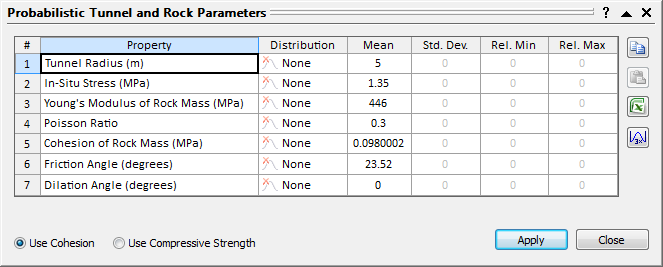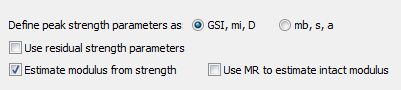# Probabilistic Input Parameters

If the Analysis Type = Probabilistic in the Project Settings dialog, then you can define statistical distributions for all of the variables in the Tunnel and Rock Parameters dialog.

To simplify the data input of statistical parameters, the Tunnel and Rock Parameters dialog will be presented in a grid format, as shown below.## Distribution

To define statistical parameters for a variable, you must first select a statistical distribution. The following distributions are available in RocSupport: Normal, Uniform, Triangular, Beta, Exponential, Lognormal and Gamma. See the individual topics for information about each distribution.

If you do not wish to define a statistical distribution for a variable, then just leave the Distribution = None. In this case, the variable will only be defined by its deterministic (Mean) value (i.e. it will be a constant for the Probabilistic analysis).

## Mean

The mean (deterministic) value of the variable.

## Standard Deviation

The standard deviation of the statistical variable. NOTE: not all distributions require the input of a standard deviation. For Uniform, Triangular and Exponential distributions, a user-defined standard deviation cannot be entered, because the standard deviation is already defined by the nature of the distribution.

## Relative Minimum and Maximum Values

It is important to note that the Minimum and Maximum values for a statistical distribution are specified as RELATIVE distances from the mean, in the Tunnel and Rock Parameters dialog. This simplifies the data input.

For example: if the Mean In-Situ Stress = 3 MPa and the actual Minimum is 2.5 MPa, then you would enter a relative Minimum = 0.5.

## Automatic Calculation of Relative Min and Max Values

For a Normal distribution, over 99% of all values should fall within 3 standard deviations of the Mean value. For convenience, after you enter the Standard Deviation for a variable you can select the button. This will automatically set the Relative Minimum and Relative Maximum Values equal to 3 times the Standard Deviation. This will ensure that a full (non-truncated) Normal distribution will be generated for the variable during the statistical sampling process.

## Dialog Options

For each Solution Method, the corresponding options (e.g. selection of cohesion or compressive strength) are displayed at the bottom left corner of the dialog. Some of these options are described below.

## Define Peak Strength Parameters

If you are using the Carranza-Torres solution method, in most cases, you will define the peak strength parameters using GSI, mi and D. In this case, the Generalized Hoek-Brown parameters mb, s and a, are automatically computed from GSI, mi and D. The Define Peak Strength As option allows you to enter the peak strength parameters directly in terms of mb, s and a.## Use Residual Strength Parameters

If you are using the Carranza-Torres, Lee and Pietruszczak, or Barbosa solution method, you may include the residual strength parameters as probabilistic analysis variables, by selecting the Use Residual Strength Parameters check box. If this check box is selected, the residual strength parameters will appear as variables in the probabilistic input dialog.